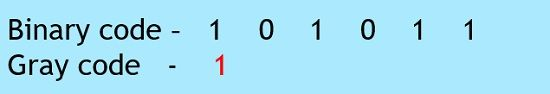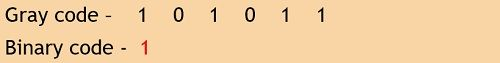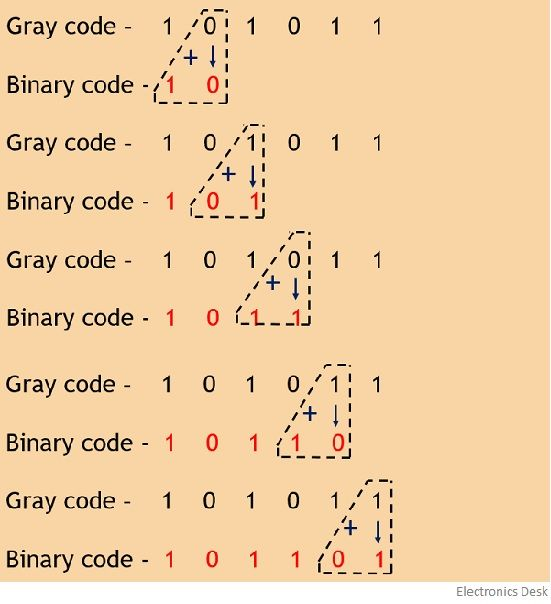# Gray Code

Definition: Gray Code is the minimum-change code category of coding in which, the two consecutive values changes by only a single bit. More specifically we can say, it is a binary number system where while moving from one step to the next, only a single bit shows variation.

This coding technique was invented by Frank Gray, thus it is named so.

It is also termed as reflected binary code or cyclic code. It is an unweighted code, as here like other number systems, no particular weight is provided to various bit positions.

Basically, binary code is changed to gray equivalent in order to lessen the switching operations. As only a single bit is changed at a particular time duration this leads to a reduction in switching from one bit to another.

Let us have a look at the tabular representation, showing gray value for different binary values:

Decimal ValueBinary CodeGray Code
000000000
100010001
200100011
300110010
401000110
501010111
601100101
701110100
810001100
910011101
A10101111
B10111110
C11001010
D11011011
E11101001
F11111000

Consider decimal value 7 and 8, to understand the switching of bits.

We know in binary, 7 is written as 0111 while 8 is written as 1000. So, we can see thatThus we can conclude that in a binary system, all 4 bits are getting changed simultaneously. Hence we can say multiple bits are changing at the same time.

In gray code, 7 is written as 0100, as against 8 is written as 1100.

So, here we can see that only a single bit i.e., MSB is changing from 0 to 1 rest other bits are the same.

This shows that in binary coding, multiple bits are changing simultaneously, while in gray coding only a single bit is getting change at a time to move from one value to another.

Therefore, we can say switching is easy in gray code than in binary code.

Now, the question arises, how can we achieve, a gray equivalent from a binary code or vice versa. For this, we will separately discuss the conversion process using examples.

### Binary to Gray Code Conversion

The conversion process from binary code to a gray involves the following steps:

1. Firstly, record the most significant bit or MSB or the leftmost bit of the given binary data as it is, to have MSB of gray equivalent.
2. Now, proceed towards adding the adjacent bits of the binary data starting from MSB with its adjacent bit to LSB. While adding, put the summation obtained in place of next bit and ignoring the carry.
3. Repeat the same process for all the bits in the sequence till LSB.

This is how the binary code is converted into gray equivalent.

• Let us take some examples to understand the above-discussed steps clearly.

(110101)2

Suppose this is the binary value which is to be converted into equivalent gray value.

As we have discussed while mentioning the steps, that the first bit or MSB of the gray equivalent will be the same as the MSB of the binary value.

ThusNow add the two adjacent bits starting from MSB to LSB and writing the result obtained as the next bit.We know the addition of binary 1 and 1 will give 0 as the sum and 1 as the carry. And we have already discussed that the carry bit must be ignored, while the sum bit achieved will be put as the next bit in the gray value.

Further, repeating the same process,So, the equivalent gray value for the given binary code is given as

(101111)Gray

• Let us take another example to have a better understanding of the same.

(101011)2

Consider the above given binary value which is to be converted into gray equivalent.

So, first, we will write the MSB of the binary digit as the MSB for the gray equivalent.

ThusNow start adding the adjacent terms of the binary code in order to get the gray codeHence the gray code for the above-given binary code will be

(111110)Gray

So, in this way a binary code is converted into gray equivalent.

Let us now understand how gray code is converted into binary code.

### Gray to Binary Code Conversion

The steps given below are required to be followed in order to convert a given gray value into its binary equivalent:

1. Like in case of binary to gray conversion, here also while writing binary code from gray code, the MSB must remain the same. So, write the leftmost bit of gray code as the MSB of binary code.
2. Now, add the recently achieved binary digit with the next adjacent gray code bit. The sum must be written as the next bit of binary equivalent, while the carry must be neglected.
3. The above-discussed step must be followed for all the bits present in the sequence.

In this way, a gray code is converted into the binary equivalent.

• Let us proceed towards some examples:

(101011)Gray

So, in the first step, writing the MSB of gray value as the MSB of binary equivalent.Now on adding the achieved binary bit and the next adjacent gray bit till the LSB of the sequence,(101101)2

• Now have a look at one more example,

(111100)Gray

Again writing the first bit of binary equivalent as the first digit of the gray codeNow on adding the binary code with the gray value, we will get,Thus the equivalent binary code will be

(101000)2

#### Applications of Gray Code

• Due to switching of a single bit, error correction can be easily achieved. Thus used in digital communication schemes such as cable TV etc.
• Also finds applications in shaft encoders, as in this possibility of errors increases with variation in the number of bits.

In this way binary to gray code and gray to binary code conversion is performed.